Anzeige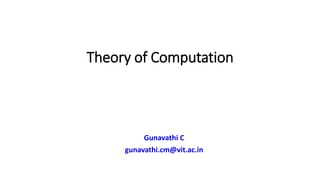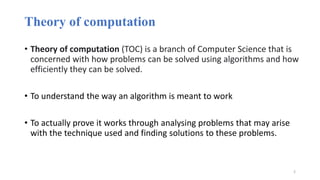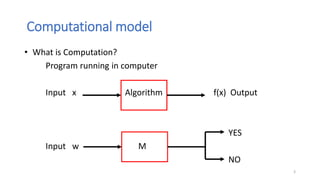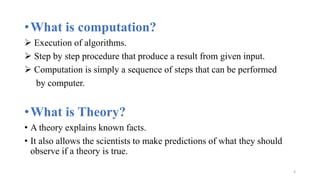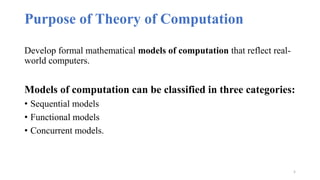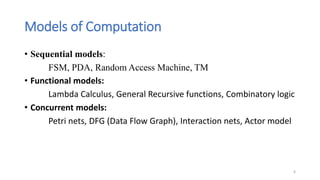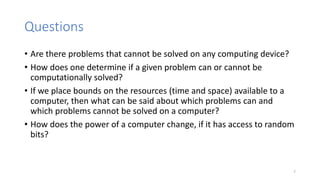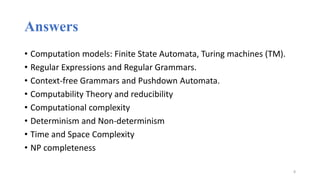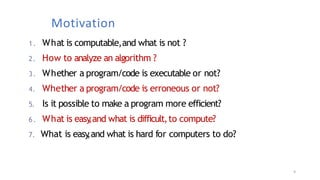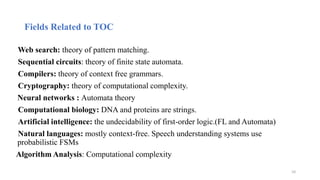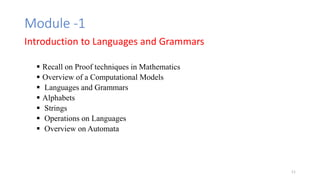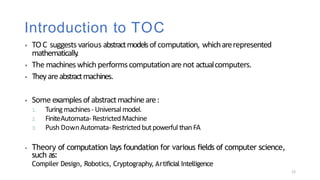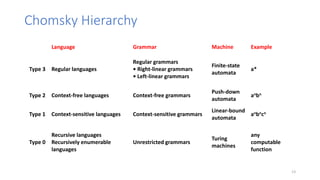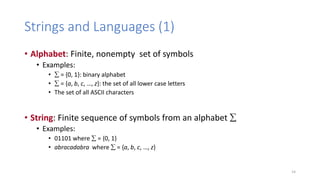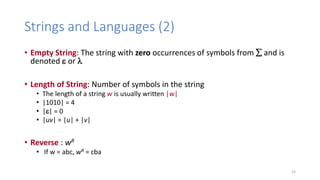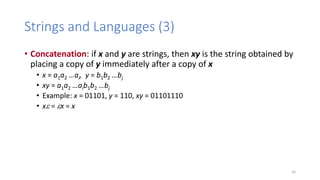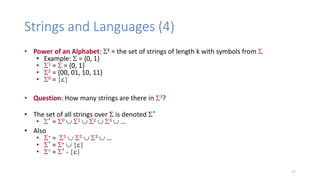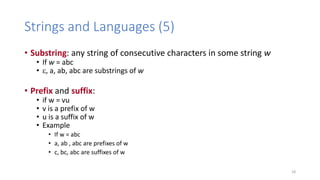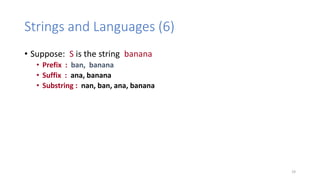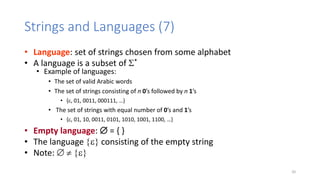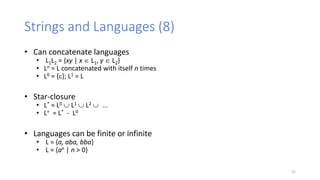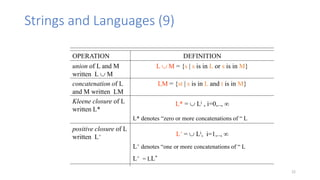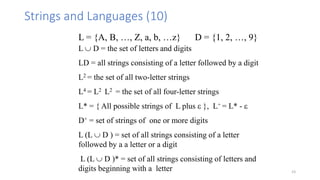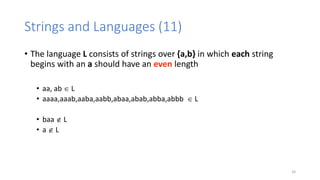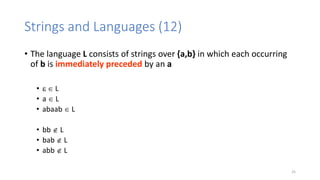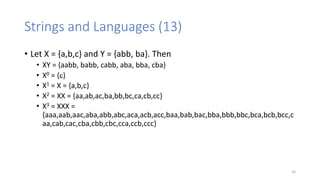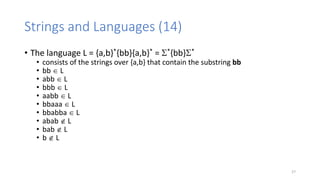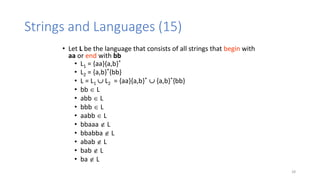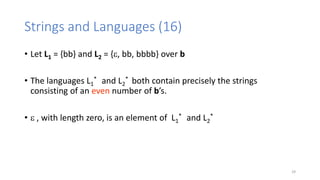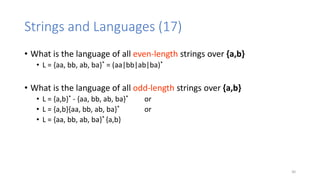1 von 30
Anzeige

### Unit-1-part-1.pptx

1. Theory of Computation Gunavathi C gunavathi.cm@vit.ac.in
2. Theory of computation • Theory of computation (TOC) is a branch of Computer Science that is concerned with how problems can be solved using algorithms and how efficiently they can be solved. • To understand the way an algorithm is meant to work • To actually prove it works through analysing problems that may arise with the technique used and finding solutions to these problems. 2
3. Computational model • What is Computation? Program running in computer Input x Algorithm f(x) Output YES Input w M NO 3
4. •What is computation?  Execution of algorithms.  Step by step procedure that produce a result from given input.  Computation is simply a sequence of steps that can be performed by computer. •What is Theory? • A theory explains known facts. • It also allows the scientists to make predictions of what they should observe if a theory is true. 4
5. Purpose of Theory of Computation Develop formal mathematical models of computation that reflect real- world computers. Models of computation can be classified in three categories: • Sequential models • Functional models • Concurrent models. 5
6. Models of Computation • Sequential models: FSM, PDA, Random Access Machine, TM • Functional models: Lambda Calculus, General Recursive functions, Combinatory logic • Concurrent models: Petri nets, DFG (Data Flow Graph), Interaction nets, Actor model 6
7. Questions • Are there problems that cannot be solved on any computing device? • How does one determine if a given problem can or cannot be computationally solved? • If we place bounds on the resources (time and space) available to a computer, then what can be said about which problems can and which problems cannot be solved on a computer? • How does the power of a computer change, if it has access to random bits? 7
8. Answers • Computation models: Finite State Automata, Turing machines (TM). • Regular Expressions and Regular Grammars. • Context-free Grammars and Pushdown Automata. • Computability Theory and reducibility • Computational complexity • Determinism and Non-determinism • Time and Space Complexity • NP completeness 8
9. Motivation 1. What is computable,and what is not ? 2. How to analyze an algorithm ? 3. Whether a program/code is executable or not? 4. Whether a program/code is erroneous or not? 5. Is it possible to make a program more efficient? 6. What is easy ,and what is difficult,to compute? 7. What is easy ,and what is hard for computers to do? 9
10. Fields Related to TOC Web search: theory of pattern matching. Sequential circuits: theory of finite state automata. Compilers: theory of context free grammars. Cryptography: theory of computational complexity. Neural networks : Automata theory Computational biology: DNA and proteins are strings. Artificial intelligence: the undecidability of first-order logic.(FL and Automata) Natural languages: mostly context-free. Speech understanding systems use probabilistic FSMs Algorithm Analysis: Computational complexity 10
11. Module -1 Introduction to Languages and Grammars  Recall on Proof techniques in Mathematics  Overview of a Computational Models  Languages and Grammars  Alphabets  Strings  Operations on Languages  Overview on Automata 11
12. Introduction to TOC ⦁ TOC suggests various abstractmodelsofcomputation, whicharerepresented mathematically . ⦁ The machineswhich performscomputationarenot actualcomputers. ⦁ Theyareabstractmachines. ⦁ Some examplesof abstractmachineare: 1. Turingmachines- Universalmodel. 2. FiniteAutomata- RestrictedMachine 3. Push DownAutomata-Restrictedbutpowerful thanFA ⦁ Theory of computation lays foundation for various fields of computer science, such as: Compiler Design, Robotics, Cryptography, Artificial Intelligence 12
13. Chomsky Hierarchy Language Grammar Machine Example Type 3 Regular languages Regular grammars • Right-linear grammars • Left-linear grammars Finite-state automata a* Type 2 Context-free languages Context-free grammars Push-down automata anbn Type 1 Context-sensitive languages Context-sensitive grammars Linear-bound automata anbncn Type 0 Recursive languages Recursively enumerable languages Unrestricted grammars Turing machines any computable function 13
14. Strings and Languages (1) • Alphabet: Finite, nonempty set of symbols • Examples: •  = {0, 1}: binary alphabet •  = {a, b, c, …, z}: the set of all lower case letters • The set of all ASCII characters • String: Finite sequence of symbols from an alphabet  • Examples: • 01101 where  = {0, 1} • abracadabra where  = {a, b, c, …, z} 14
15. Strings and Languages (2) • Empty String: The string with zero occurrences of symbols from  and is denoted e or  • Length of String: Number of symbols in the string • The length of a string w is usually written |w| • |1010| = 4 • |e| = 0 • |uv| = |u| + |v| • Reverse : wR • If w = abc, wR = cba 15
16. Strings and Languages (3) • Concatenation: if x and y are strings, then xy is the string obtained by placing a copy of y immediately after a copy of x • x = a1a2 …ai, y = b1b2 …bj • xy = a1a2 …aib1b2 …bj • Example: x = 01101, y = 110, xy = 01101110 • xe = ex = x 16
17. Strings and Languages (4) • Power of an Alphabet: k = the set of strings of length k with symbols from  • Example:  = {0, 1} • 1 =  = {0, 1} • 2 = {00, 01, 10, 11} • 0 = {e} • Question: How many strings are there in 3? • The set of all strings over  is denoted * • * = 0  1  2  3  … • Also • + = 1  2  3  … • * = +  {e} • + = * - {e} 17
18. Strings and Languages (5) • Substring: any string of consecutive characters in some string w • If w = abc • e, a, ab, abc are substrings of w • Prefix and suffix: • if w = vu • v is a prefix of w • u is a suffix of w • Example • If w = abc • a, ab , abc are prefixes of w • c, bc, abc are suffixes of w 18
19. Strings and Languages (6) • Suppose: S is the string banana • Prefix : ban, banana • Suffix : ana, banana • Substring : nan, ban, ana, banana 19
20. Strings and Languages (7) • Language: set of strings chosen from some alphabet • A language is a subset of * • Example of languages: • The set of valid Arabic words • The set of strings consisting of n 0’s followed by n 1’s • {e, 01, 0011, 000111, …} • The set of strings with equal number of 0’s and 1’s • {e, 01, 10, 0011, 0101, 1010, 1001, 1100, …} • Empty language:  = { } • The language {e} consisting of the empty string • Note:   {e} 20
21. Strings and Languages (8) • Can concatenate languages • L1L2 = {xy | x  L1, y  L2} • Ln = L concatenated with itself n times • L0 = {e}; L1 = L • Star-closure • L* = L0  L1  L2  ... • L+ = L* - L0 • Languages can be finite or infinite • L = {a, aba, bba} • L = {an | n > 0} 21
22. Strings and Languages (9) OPERATION DEFINITION union of L and M written L  M concatenation of L and M written LM Kleene closure of L written L* positive closure of L written L+ L  M = {s | s is in L or s is in M} LM = {st | s is in L and t is in M} L+ =  Li, i=1,..,  L* denotes “zero or more concatenations of “ L L* =  Li , i=0,..,  L+ denotes “one or more concatenations of “ L L+ = LL* 22
23. Strings and Languages (10) L = {A, B, …, Z, a, b, …z} D = {1, 2, …, 9} L  D = the set of letters and digits LD = all strings consisting of a letter followed by a digit L2 = the set of all two-letter strings L4 = L2 L2 = the set of all four-letter strings L* = { All possible strings of L plus e }, L+ = L* - e D+ = set of strings of one or more digits L (L  D ) = set of all strings consisting of a letter followed by a a letter or a digit L (L  D )* = set of all strings consisting of letters and digits beginning with a letter 23
24. Strings and Languages (11) • The language L consists of strings over {a,b} in which each string begins with an a should have an even length • aa, ab  L • aaaa,aaab,aaba,aabb,abaa,abab,abba,abbb  L • baa  L • a  L 24
25. Strings and Languages (12) • The language L consists of strings over {a,b} in which each occurring of b is immediately preceded by an a • e  L • a  L • abaab  L • bb  L • bab  L • abb  L 25
26. Strings and Languages (13) • Let X = {a,b,c} and Y = {abb, ba}. Then • XY = {aabb, babb, cabb, aba, bba, cba} • X0 = {e} • X1 = X = {a,b,c} • X2 = XX = {aa,ab,ac,ba,bb,bc,ca,cb,cc} • X3 = XXX = {aaa,aab,aac,aba,abb,abc,aca,acb,acc,baa,bab,bac,bba,bbb,bbc,bca,bcb,bcc,c aa,cab,cac,cba,cbb,cbc,cca,ccb,ccc} 26
27. Strings and Languages (14) • The language L = {a,b}*{bb}{a,b}* = *{bb}* • consists of the strings over {a,b} that contain the substring bb • bb  L • abb  L • bbb  L • aabb  L • bbaaa  L • bbabba  L • abab  L • bab  L • b  L 27
28. Strings and Languages (15) • Let L be the language that consists of all strings that begin with aa or end with bb • L1 = {aa}{a,b}* • L2 = {a,b}*{bb} • L = L1  L2 = {aa}{a,b}*  {a,b}*{bb} • bb  L • abb  L • bbb  L • aabb  L • bbaaa  L • bbabba  L • abab  L • bab  L • ba  L 28
29. Strings and Languages (16) • Let L1 = {bb} and L2 = {e, bb, bbbb} over b • The languages L1 * and L2 * both contain precisely the strings consisting of an even number of b’s. • e , with length zero, is an element of L1 * and L2 * 29
30. Strings and Languages (17) • What is the language of all even-length strings over {a,b} • L = {aa, bb, ab, ba}* = (aa|bb|ab|ba)* • What is the language of all odd-length strings over {a,b} • L = {a,b}* - {aa, bb, ab, ba}* or • L = {a,b}{aa, bb, ab, ba}* or • L = {aa, bb, ab, ba}* {a,b} 30
Anzeige